# DISCOUNT - Ticket Total - Ask Fixed Amount

This method allows for a Discount to be applied to the Ticket Total, based on a choice of 5 different fixed amounts.  It uses an Ask/Apply approach using the `Ask Question` Action to let the user choose an amount, then applies the Discount using a `Update Calculation` Action.

Operational Flow:

Calculation Type: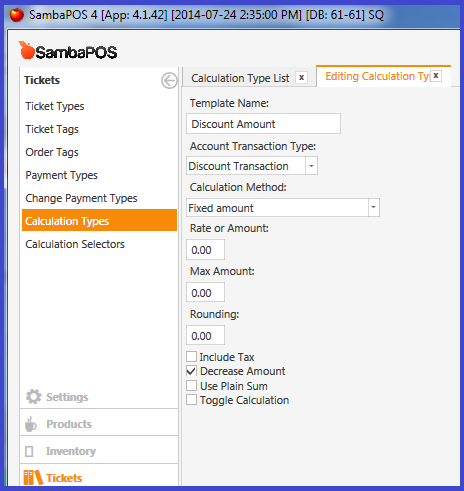Automation Command - Apply: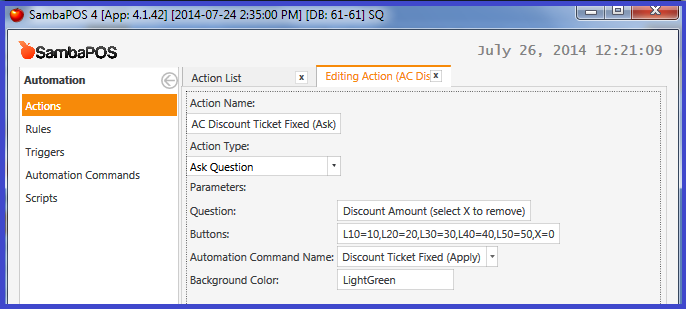Action - Update Ticket Calculation: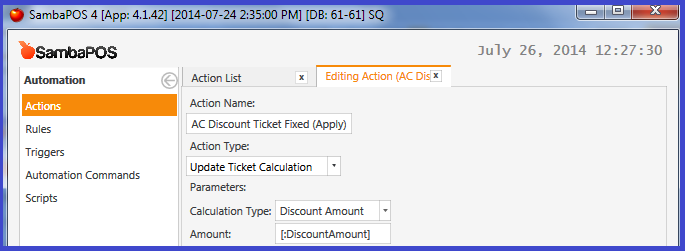Rule - Apply Discount:

2 Likes

Is any method allows for a Discount Percent to be applied to Ticket Total based on user input of a Percentage.

Yes…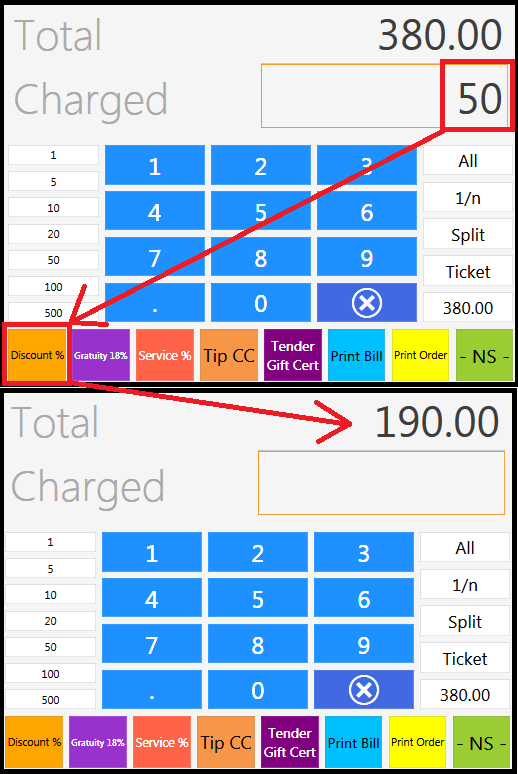how can i do a fixed percentage discount on a total amount so lets say \$10 you get 1% on \$50 you get 5% and so on?

@qmckay i like the post in my scenario i need a discount ask amount example a ticket total is 45 i need to take 25 so i need a discount to ask the amount if i enter 25 than the ticket price will update to 25 rest of the amount is discount any suggetions help on this would be a great help thanks

Tickets->Calculation Types-> Discount-> Calculation Method-> Rate from ticket amount

IN CUSTOM REPORT

``````[DISCOUNT:2, 2, 2]
{REPORT CALCULATION DETAILS:T.Date,EN.Masalar,T.TotalAmount}
``````

and how to show “how many %”

IN CUSTOM REPORT

``````[DISCOUNT:2, 2, 2]
{REPORT CALCULATION DETAILS:T.Date,EN.Masalar,T.TotalAmount}
``````

and how to show “how many %”

I have a problem, when I put the mapping on to “payment”, I need to hit close, and after hit “SETTLE” so I can see the Discount Amount

Thanks.

Execute `Display Payment Screen` action to refresh payment screen.

2 Likes

It works.

1000 yıl yaşamak için1 Like

Hey again,

To take the % from the total should be like this: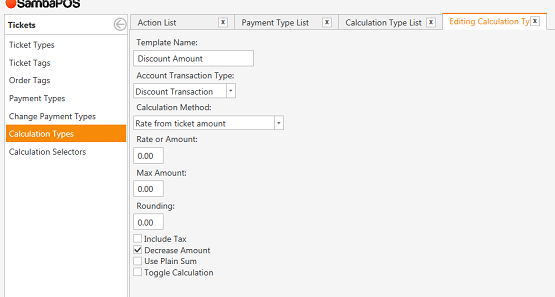Thanks

Thanks